Like   Tweet   +1
 /* styles */ Issue 63, March 2019

## Wha gwaan?

 /* styles */ Plenty. Dat wha gwaan. Easter is just around the corner. For some that means eating too much chocolate. For others a State Championship. For me it's both which may explain the likely result. There are dates for your calendar and other genuinely important info. Plus my contributions, that even the front half of Da Easy Crew thought somewhat random. Clicked links will make sense of it all though. Let's start with a couple of items I need you to look at. Then, well, we will see how it goes. Good luck...

## Club & State Race Officer Courses

There's still time to get involved, just. We would be grateful if someone would take on the State Race Officer course and be willing to run events next year.

 /* styles */ Boyd Newton, Da Easy Crew, Editor.
 table div table+table+table+table+table+table+table div table{width:100%;padding:0}table div table+table+table+table+table+table+table div table img{width:96.23%;padding:0;float:none}table div table+table+table+table+table+table+table div table td{width:100%;padding:0 1.88% 18px}/* styles */## Important Info

Please look into the following as others may be relying on you to do so:
* Easter Egg Hunt
* Race Officer Courses
* Presentation Night
* Notice to Powerboat Crews

## Dubious Info

 /* styles */ I was having trouble with the end of ropes fraying. I asked Gerald Casale, Bob Lewis, Mark Mothersbaugh, Bob Casale, Rod Reisman and Fred Weber if they had any tips for me. They all had the same advice when a problem comes along...
 table div table+table+table+table+table+table+table+table+table+table+table div table{width:100%;padding:0}table div table+table+table+table+table+table+table+table+table+table+table div table img{width:96.23%;padding:0;float:none}table div table+table+table+table+table+table+table+table+table+table+table div table td{width:100%;padding:0 1.88% 18px}/* styles */## A picture tells a thousand words

I thought to myself, have a quick look through the BSC photos. That was several hours ago. It's now 1:17am and I'm no closer to a favourite. I didn't struggle to find some spectacular shots - quite the opposite - there's just too many!

## Who's to blame for this?

Rob Owe-Young. Come rain or shine, Rob & Karen (thanks for snapping the photographer Karen) head out on the powerboats to capture the most amazing images of us.On auto. May expose himself.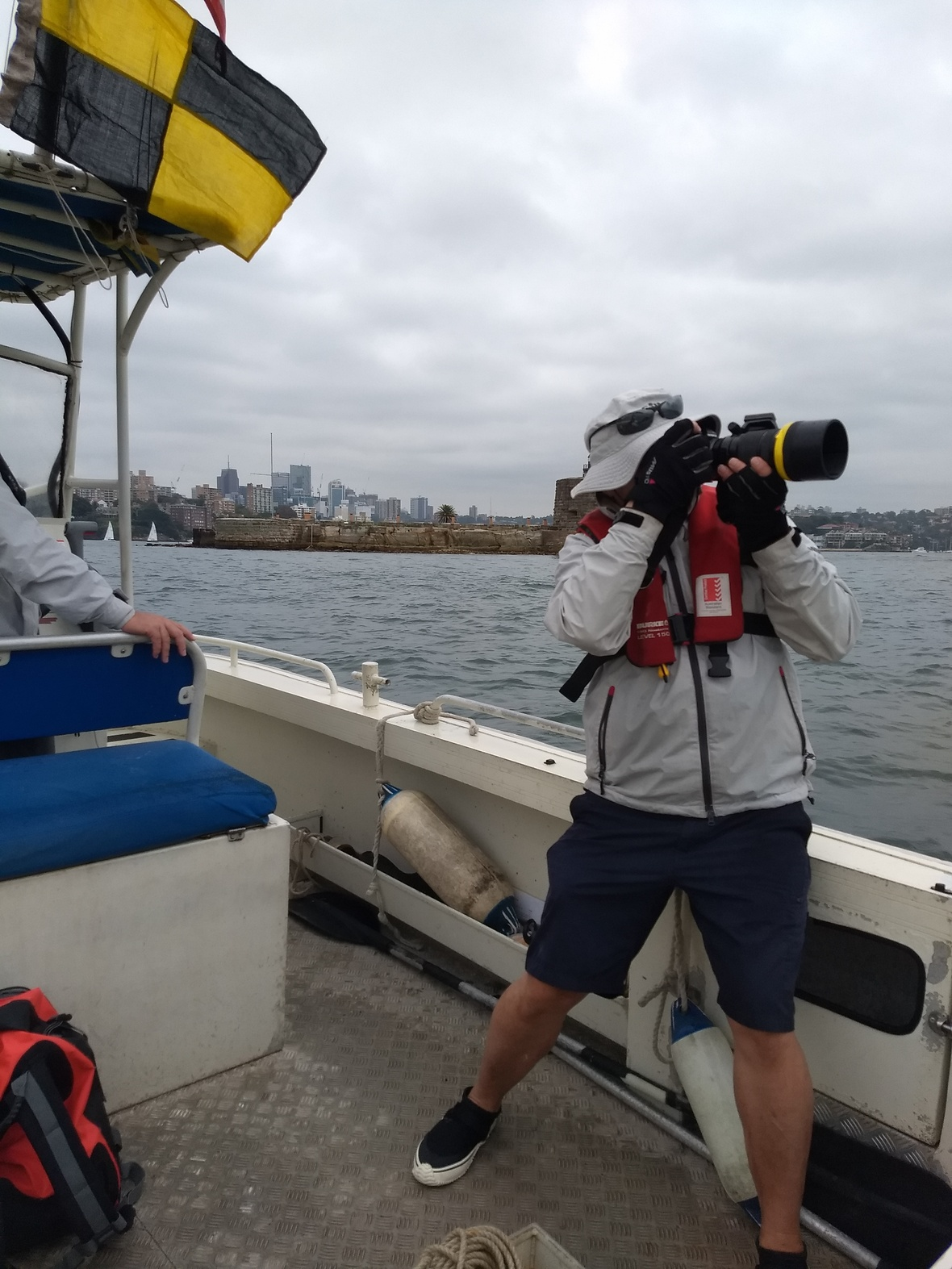Capturing the moment.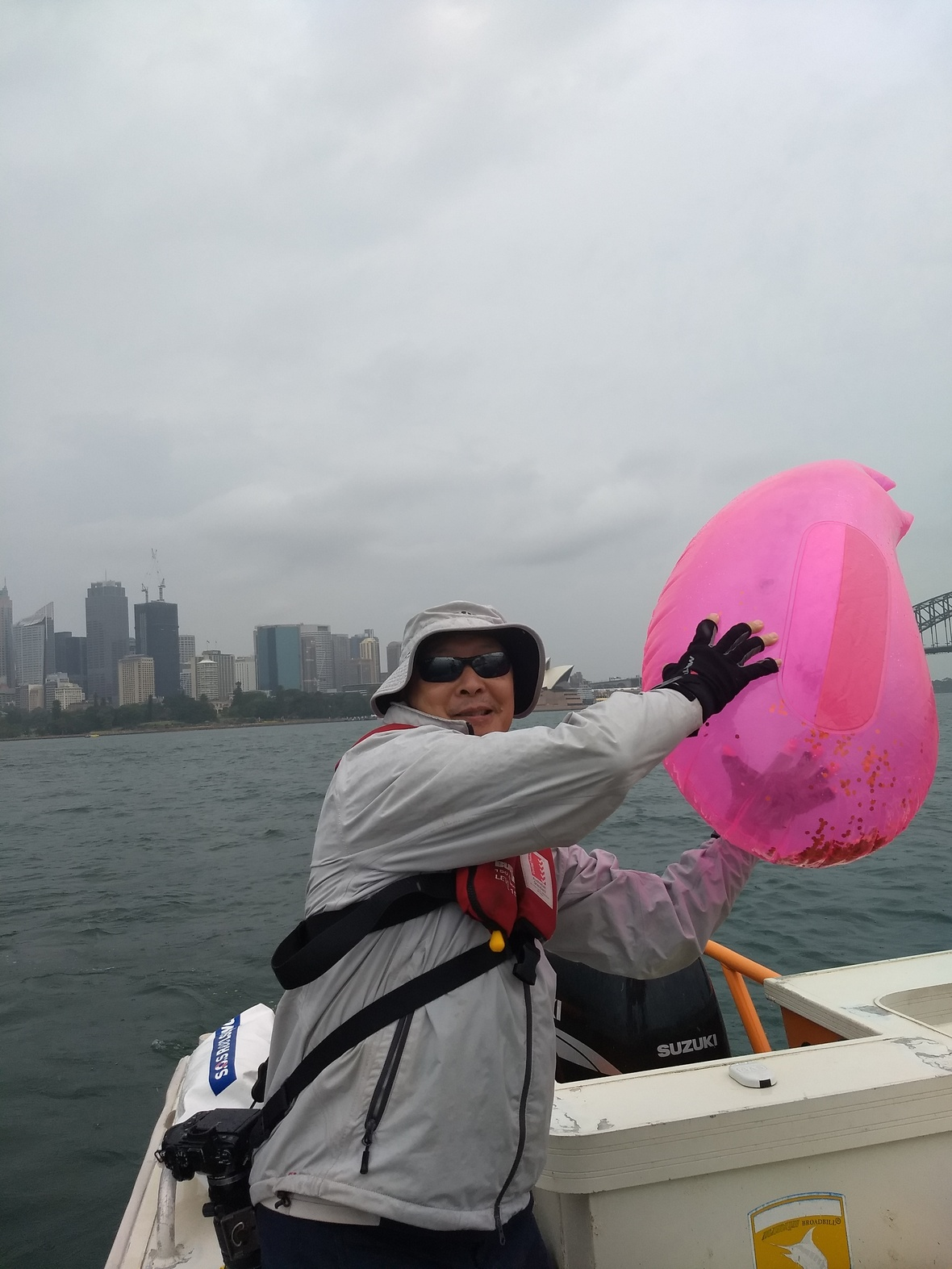Capturing who knows what?
 /* styles */ Everyone talks about your photos and looks forward to seeing what moments have been immortalised each week. It is really appreciated. You may not see Rob around the Club, he will be lurking in the shadows (making sure the light is correct) behind a lens. But if you catch a glimpse, then let him know your favourite photo and say thanks.
 /* styles */ For me, perhaps the one where we just rounded 6 and More Edge in hot pursuit launched off a wave, almost plummeting down into the back of my head. I say perhaps, because I can't even find it, let alone compare it with all the others - Thanks!
 table div table+table+table+table+table+table+table+table+table+table+table+table+table+table+table+table+table div table{width:100%;padding:0}table div table+table+table+table+table+table+table+table+table+table+table+table+table+table+table+table+table div table img{width:96.23%;padding:0;float:none}table div table+table+table+table+table+table+table+table+table+table+table+table+table+table+table+table+table div table td{width:100%;padding:0 1.88% 18px}/* styles */## Easter Egg Hunt

 /* styles */ April 13th will see hoards of little maniacs running around the Balmoral foreshore wreaking havoc. So what's different to any other Saturday? That week they have an excuse.
 table div table+table+table+table+table+table+table+table+table+table+table+table+table+table+table+table+table+table+table+table div table td,table.module-19{width:100%;padding:0}table div table+table+table+table+table+table+table+table+table+table+table+table+table+table+table+table+table+table+table+table div table{width:100%;float:none;margin-left:auto;margin-right:auto;padding:0}table div table+table+table+table+table+table+table+table+table+table+table+table+table+table+table+table+table+table+table+table div table a{border:0 none;text-decoration:none}table div table+table+table+table+table+table+table+table+table+table+table+table+table+table+table+table+table+table+table+table div table img{width:100%!important;border:0 none;text-decoration:none}/* styles */
 /* styles */ While you shouldn't eat everything brown they drop, you can't have Easter without the rabbit rabbit rabbit rabbit..!
 table div table+table+table+table+table+table+table+table+table+table+table+table+table+table+table+table+table+table+table+table+table+table+table div table{width:100%;padding:0}table div table+table+table+table+table+table+table+table+table+table+table+table+table+table+table+table+table+table+table+table+table+table+table div table img{width:96.23%;padding:0;float:none}table div table+table+table+table+table+table+table+table+table+table+table+table+table+table+table+table+table+table+table+table+table+table+table div table td{width:100%;padding:0 1.88% 18px}/* styles */## Aero News

 /* styles */ The RS Aero Victorian State Championships 2019 were held at Mount Martha Yacht Club over March 9, 10 and 11. The series had a total of twenty-five entries with 20 sailors from Victoria, 4 from NSW and 1 from SA competing in the 5, 7 and 9 rigs. Michael Savery and Nick Collis-George represented Balmoral SC. Seven of the 10 programmed races were sailed in light, moderate and heavy conditions. The first day started with a slight breeze and a smoke covered Port Phillip Bay. The afternoon brought champagne conditions with two races sailed in eight to 12 knot south westerly breeze.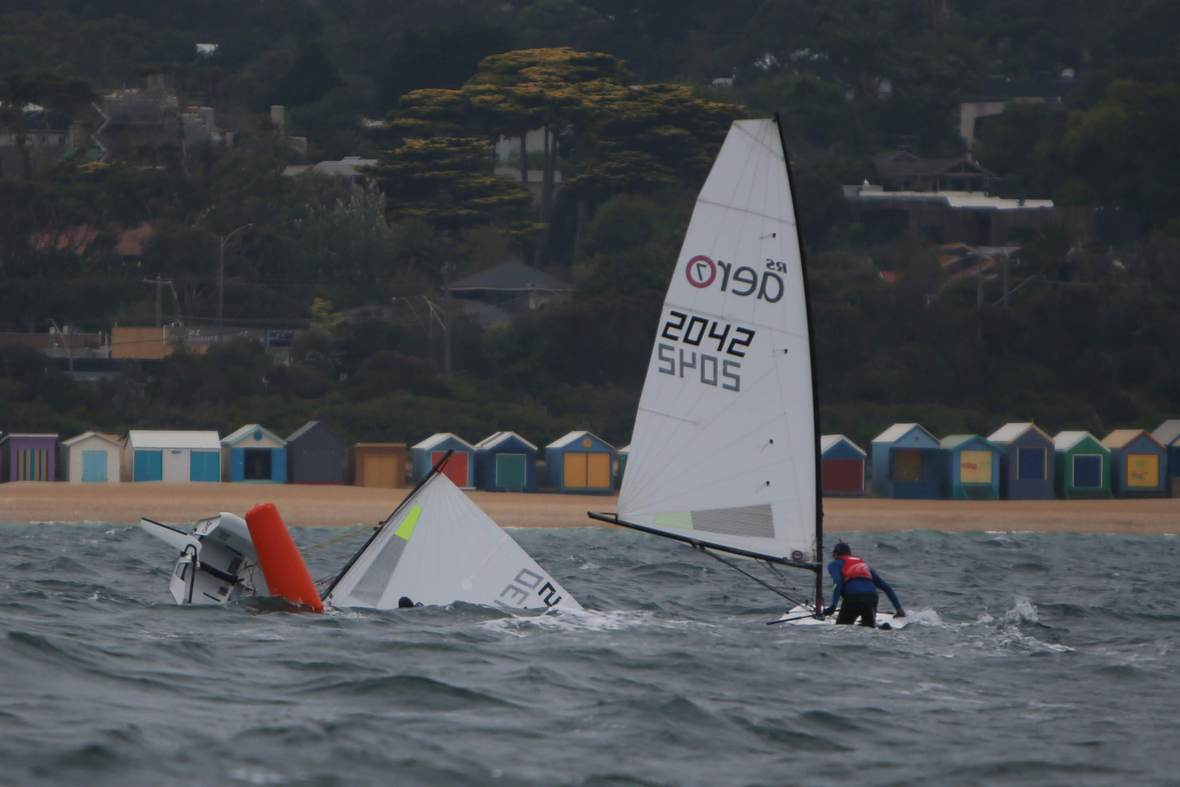Michael approaches bottom mark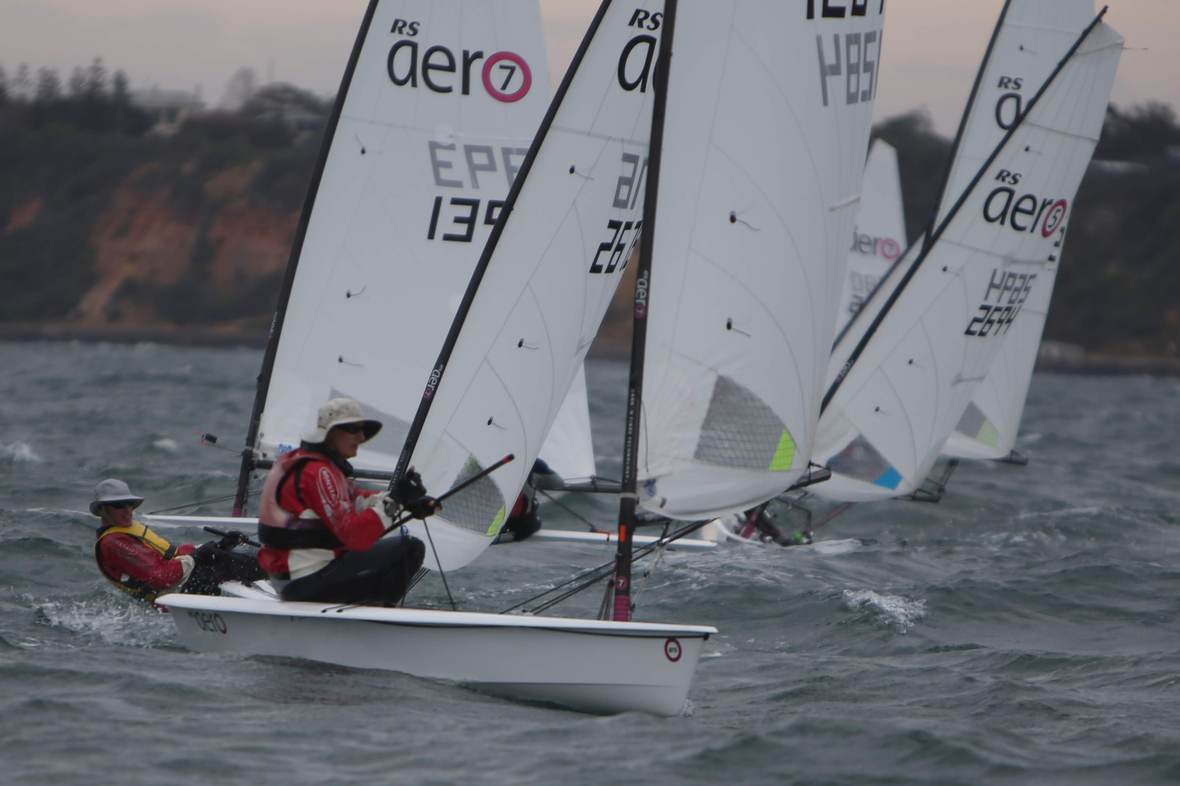Nick leads at the top mark
 /* styles */ Day two built to a solid 18 to 22 knot south-westerly and 3 races completed. With triangular courses set there were spectacular reaches and plenty of surfing, with plenty of centreboards coming up for air at the gybe mark. In the 7 rigs Rhett Gowans led the regatta before breaking his tiller, opening the door for both Nick Collis-George and Chris Brain to lead the 7s home. The last day was light and lumpy with left over sea from the night before, about 8-12 knots and a mix of overcast sky, heavy rain and bright sunshine. MMYC, a volunteer only club, put on an excellent regatta. In the 7 Rig, BSC sailors Michael Savery came 5th, and Nick Collis-George 2nd. Nick Collis-George, Aero Class Captain & Director, BSC
 table div table+table+table+table+table+table+table+table+table+table+table+table+table+table+table+table+table+table+table+table+table+table+table+table+table+table+table+table div table{width:100%;padding:0}table div table+table+table+table+table+table+table+table+table+table+table+table+table+table+table+table+table+table+table+table+table+table+table+table+table+table+table+table div table img{width:96.23%;padding:0;float:none}table div table+table+table+table+table+table+table+table+table+table+table+table+table+table+table+table+table+table+table+table+table+table+table+table+table+table+table+table div table td{width:100%;padding:0 1.88% 18px}/* styles */## TANSW - Training Days

Ahead of the State Championships there's a training day at George’s River Sailing Club. The day will be a mix of coaching and racing, under the keen instruction & sharing of knowledge from current Tasar champions.
Details:
Date: Sunday 14th April
Venue: GRSC
Time: 9am-5pm
RSVP: Jimmy Burman jweburman@gmail.com or 0439 639 031

BSC will hold another training day after the States, before boats are loaded into containers for the World Titles. We are looking at the 11th of May with training built around our Winter Sprints. Details to be confirmed.

## NSW States - Easter Long Weekend - GRSC

The Tasar Association of New South Wales Incorporated (TANSW) in conjunction with George’s River Sailing Club invite us to the 42nd New South Wales Tasar Championship, to be held on the waters of Botany Bay from 19th April to 21st April, 2019.

Want to compete but something is stopping you?

Let us know as we may be able to help.

## World Titles - 27th July, 2019 - Hayling Island, UK

Containers will be packed on May 25th for those going to the 2019 Tasar World Championships being held at Hayling Island Sailing Club.

It's not to late to get involved as according to Google Maps it's only 1h 25m drive from Hayling Island to Balmoral.

## Jib Sheeting Trial

Many thanks to Brad Stephens for both rejuvenating our club Tasar 'Sticky'. Brad also designed and fitted the jib sheeting trial rig which is under evaluation.

We need feedback from as many crews as possible before results can be presented to the WTC.

 /* styles */ Boyd Newton, Tasar Class Captain, BSC
 table div table+table+table+table+table+table+table+table+table+table+table+table+table+table+table+table+table+table+table+table+table+table+table+table+table+table+table+table+table+table+table+table div table{width:100%;padding:0}table div table+table+table+table+table+table+table+table+table+table+table+table+table+table+table+table+table+table+table+table+table+table+table+table+table+table+table+table+table+table+table+table div table img{width:96.23%;padding:0;float:none}table div table+table+table+table+table+table+table+table+table+table+table+table+table+table+table+table+table+table+table+table+table+table+table+table+table+table+table+table+table+table+table+table div table td{width:100%;padding:0 1.88% 18px}/* styles */## A Little Anecdote

Illustrating the "butterfly effect" that our club has...

A long time friend, when he found out I am associated with Balmoral Sailing Club said "I learned to sail there"; then

While searching for a firm to do a construction estimate for our proposed renovations the person who answered the telephone said "I am the estimator for this company, and I learned to sail at Balmoral Sailing Club". His efforts on our behalf were above and beyond the brief we gave him; then

The salvage contractor who was engaged to re-float "Balmoral" said "I learned to sail at Balmoral when at school at Redlands".

How many others are out there???

We sometimes overlook the benefits that our "little" club has delivered to individuals and the wider community over the years. Clearly, the club has provided fond memories for many, added to which our sport promotes desirable and enduring personal traits such as sportsmanship, self discipline, teamwork, risk assessment, knowledge of the weather, practical skills, and above all the joy of sailing.

Something to be proud of, and a good reason for others to support our club.

## The Sinking of "Balmoral"

On March 16th (Saturday night) there was unusually heavy rain. We think that this is the cause of "Balmoral"' sinking since there were no identified defects in the boat or equipment that would have caused its demise. Simply put, the rain was so overwhelming that even the buoyancy of the enclosed hull could not keep her afloat.

 /* styles */ We engaged a salvage contractor who re-floated "Balmoral" on Monday 18th March. She was taken to the Clontarf Marina to be put on the slip for assessment by our insurer's marine surveyor. We now await the insurer's response. A new battery and bilge pump has been installed, and you may have noticed that "Balmoral" is on her normal mooring. But nothing except the new pump works!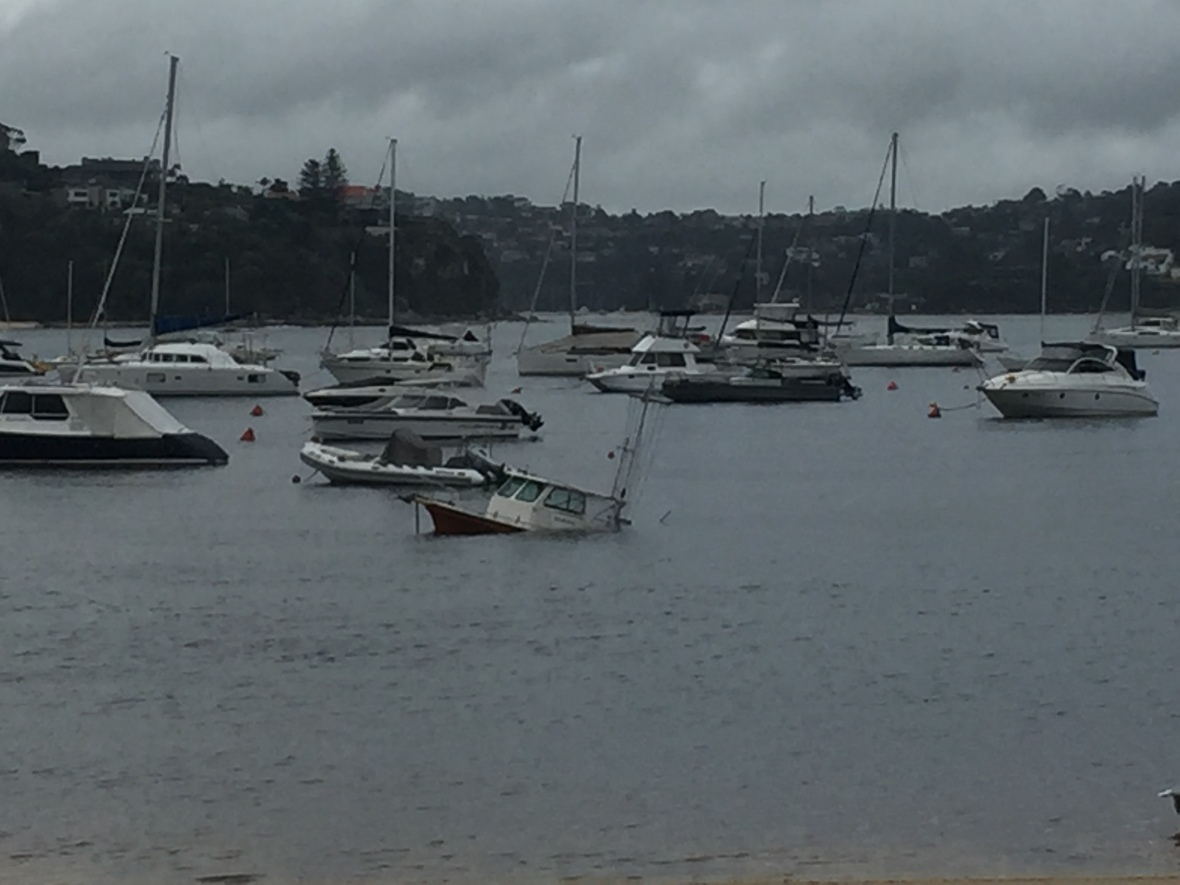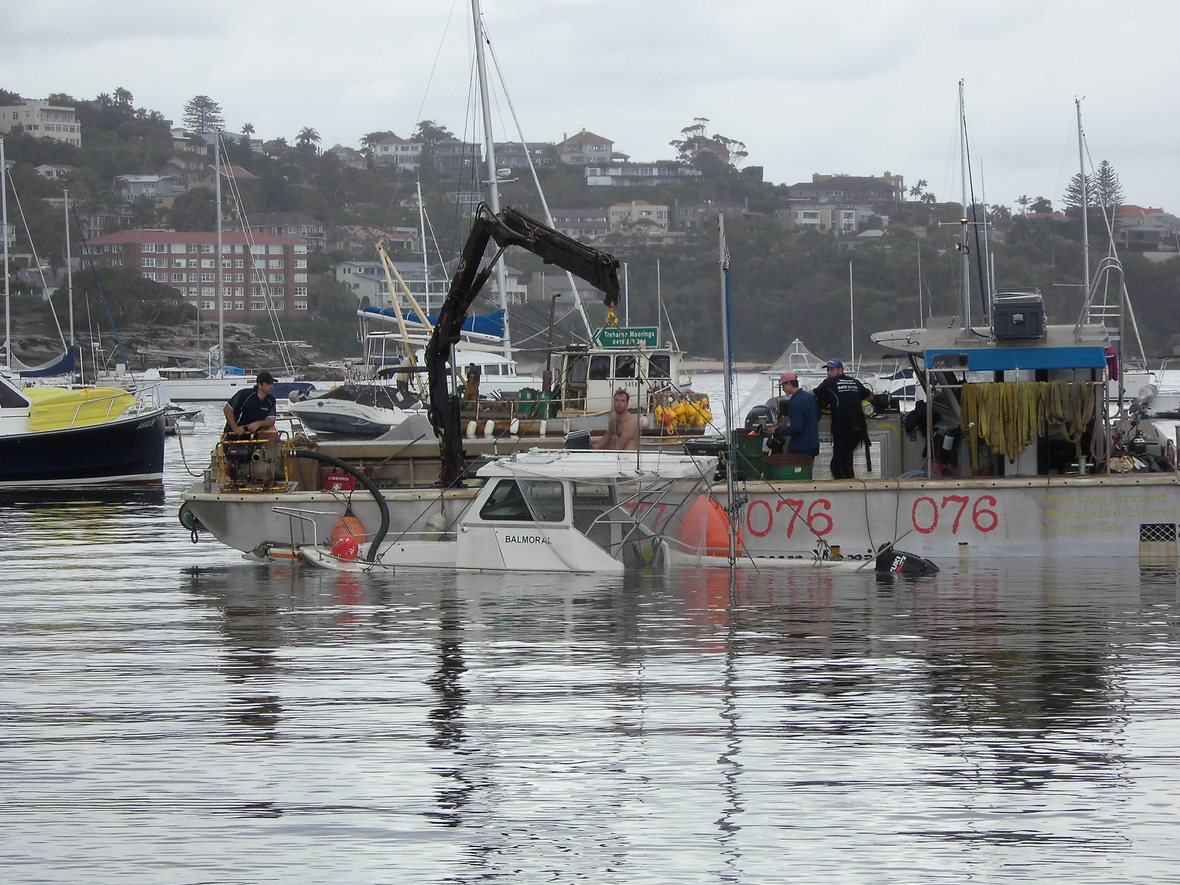/* styles */ Because of the magnitude of damage to her electrics and motor, it is unlikely that "Balmoral" will be back on the start line this season.

## Notice for the Power Boat Operators

On Saturday Roly and company in Eric Hart laid the downwind windsurfer mark about 50 metres South-West of Mark 1. No - it didn't drift away. When we came to retrieve it we found out why.

We could not get the anchor up no matter what we tried, including tying the rope off short and driving in circles around the anchor to use the motor pull at all angles. In the end we had to use motor power to break the mark rope. We got most of it back.

I have just noticed that a similar thing happened to Wes on 17 February 2018. His description was 50 metres South of Mark 1. Pretty close to the place that we had trouble.

It seems there is foul ground in this area and we should not put any marks down to the South or West of Mark 1. There be dragons that eat anchors!!!!

Roly Webb, Director, BSC

 table div table+table+table+table+table+table+table+table+table+table+table+table+table+table+table+table+table+table+table+table+table+table+table+table+table+table+table+table+table+table+table+table+table+table+table+table+table+table+table div table{width:100%;padding:0}table div table+table+table+table+table+table+table+table+table+table+table+table+table+table+table+table+table+table+table+table+table+table+table+table+table+table+table+table+table+table+table+table+table+table+table+table+table+table+table div table img{width:96.23%;padding:0;float:none}table div table+table+table+table+table+table+table+table+table+table+table+table+table+table+table+table+table+table+table+table+table+table+table+table+table+table+table+table+table+table+table+table+table+table+table+table+table+table+table div table td{width:100%;padding:0 1.88% 18px}/* styles */## Safety Audit Forms

 /* styles */ Safety audits are due so please look into your requirements. For assistance or to return once completed please see your class captains or Laurie Hoffman :-)
 table div table+table+table+table+table+table+table+table+table+table+table+table+table+table+table+table+table+table+table+table+table+table+table+table+table+table+table+table+table+table+table+table+table+table+table+table+table+table+table+table+table+table+table div table{width:100%;padding:0}table div table+table+table+table+table+table+table+table+table+table+table+table+table+table+table+table+table+table+table+table+table+table+table+table+table+table+table+table+table+table+table+table+table+table+table+table+table+table+table+table+table+table+table div table img{width:96.23%;padding:0;float:none}table div table+table+table+table+table+table+table+table+table+table+table+table+table+table+table+table+table+table+table+table+table+table+table+table+table+table+table+table+table+table+table+table+table+table+table+table+table+table+table+table+table+table+table div table td{width:100%;padding:0 1.88% 18px}/* styles */## Race Officer Training

Interested in becoming a race official?

## Club Race Officer

Training costs \$65 and is reimbursed when you volunteer as a club race officer. Conducted by Australian Sailing, the next two courses are:
* Tue 16th Apr 2019, 18:00-21:30 at Royal Prince Alfred Yacht Club
* Tue 19th Mar 2019, 18:00-21:30 at Manly 16’ Skiff Sailing Club

## State Race Officer Course

Training costs \$115 and will be reimbursed if you volunteer as a state race officer for the club. te next course is:
* 30-31 Mar 2019, 09:00-17:00 at Middle Harbour Yacht Club

Anita Daum
e: anita.daum@balmoralsc.com.au

 table div table+table+table+table+table+table+table+table+table+table+table+table+table+table+table+table+table+table+table+table+table+table+table+table+table+table+table+table+table+table+table+table+table+table+table+table+table+table+table+table+table+table+table+table+table+table div table{width:100%;padding:0}table div table+table+table+table+table+table+table+table+table+table+table+table+table+table+table+table+table+table+table+table+table+table+table+table+table+table+table+table+table+table+table+table+table+table+table+table+table+table+table+table+table+table+table+table+table+table div table img{width:96.23%;padding:0;float:none}table div table+table+table+table+table+table+table+table+table+table+table+table+table+table+table+table+table+table+table+table+table+table+table+table+table+table+table+table+table+table+table+table+table+table+table+table+table+table+table+table+table+table+table+table+table+table div table td{width:100%;padding:0 1.88% 18px}/* styles */## For Sale or Wanted

 /* styles */ Mirror Dinghy for Sale, “TRIM” - \$900 ONO Wood and Varnish finish. Gaff-rigged. Trolley, spare sails and spars. Racked at Balmoral Sailing Club. More info - click here. Contact: Matthew White on 0414 185 798
 /* styles */ Wanted, road trailer for Tasar. Contact: tasar@balmoralsc.com.au
 /* styles */ Wanted, articles for Whale's Tales - \$10/10ths of nothing Contact: Ed Itor

## Have gear for sale or looking for something specific?

Send details to e: whalestales@balmoralsc.com.au

 table div table+table+table+table+table+table+table+table+table+table+table+table+table+table+table+table+table+table+table+table+table+table+table+table+table+table+table+table+table+table+table+table+table+table+table+table+table+table+table+table+table+table+table+table+table+table+table+table+table+table+table+table div table{width:100%;padding:0}table div table+table+table+table+table+table+table+table+table+table+table+table+table+table+table+table+table+table+table+table+table+table+table+table+table+table+table+table+table+table+table+table+table+table+table+table+table+table+table+table+table+table+table+table+table+table+table+table+table+table+table+table div table img{width:96.23%;padding:0;float:none}table div table+table+table+table+table+table+table+table+table+table+table+table+table+table+table+table+table+table+table+table+table+table+table+table+table+table+table+table+table+table+table+table+table+table+table+table+table+table+table+table+table+table+table+table+table+table+table+table+table+table+table+table div table td{width:100%;padding:0 1.88% 18px}/* styles */table div table+table+table+table+table+table+table+table+table+table+table+table+table+table+table+table+table+table+table+table+table+table+table+table+table+table+table+table+table+table+table+table+table+table+table+table+table+table+table+table+table+table+table+table+table+table+table+table+table+table+table+table+table div table td,table.module-52{width:100%;padding:0}table div table+table+table+table+table+table+table+table+table+table+table+table+table+table+table+table+table+table+table+table+table+table+table+table+table+table+table+table+table+table+table+table+table+table+table+table+table+table+table+table+table+table+table+table+table+table+table+table+table+table+table+table+table div table{width:100%;float:none;margin-left:auto;margin-right:auto;padding:0}table div table+table+table+table+table+table+table+table+table+table+table+table+table+table+table+table+table+table+table+table+table+table+table+table+table+table+table+table+table+table+table+table+table+table+table+table+table+table+table+table+table+table+table+table+table+table+table+table+table+table+table+table+table div table a{border:0 none;text-decoration:none}table div table+table+table+table+table+table+table+table+table+table+table+table+table+table+table+table+table+table+table+table+table+table+table+table+table+table+table+table+table+table+table+table+table+table+table+table+table+table+table+table+table+table+table+table+table+table+table+table+table+table+table+table+table div table img{width:100%!important;border:0 none;text-decoration:none}/* styles */
 table div table+table+table+table+table+table+table+table+table+table+table+table+table+table+table+table+table+table+table+table+table+table+table+table+table+table+table+table+table+table+table+table+table+table+table+table+table+table+table+table+table+table+table+table+table+table+table+table+table+table+table+table+table+table+table div table{width:100%;padding:0}table div table+table+table+table+table+table+table+table+table+table+table+table+table+table+table+table+table+table+table+table+table+table+table+table+table+table+table+table+table+table+table+table+table+table+table+table+table+table+table+table+table+table+table+table+table+table+table+table+table+table+table+table+table+table+table div table img{width:96.23%;padding:0;float:none}table div table+table+table+table+table+table+table+table+table+table+table+table+table+table+table+table+table+table+table+table+table+table+table+table+table+table+table+table+table+table+table+table+table+table+table+table+table+table+table+table+table+table+table+table+table+table+table+table+table+table+table+table+table+table+table div table td{width:100%;padding:0 1.88% 18px}/* styles */## Ides Tides

You have likely heard of the Ides of March. And may have been warned of them. But what's it all about?

The Romans did not number days of a month from the first to the last day, instead counting back from three fixed points:

▪ the Nones - 5th or 7th, nine days inclusive before the Ides
▪ the Ides - 13th for most, but the 15th in March, May, July, and October
▪ the Kalends - 1st of the following month
 ▪ the Nones - 5th or 7th, nine days inclusive before the Ides
 ▪ the Ides - 13th for most, but the 15th in March, May, July, and October
 ▪ the Kalends - 1st of the following month

Originally the Ides were determined by the full moon, reflecting the lunar origin of the Roman calendar. In the earliest calendar, the Ides of March would have been the first full moon of the new year.

Romans also considered the Ides a deadline for settling debts. If I had forgotten to service the loan for a Tasar back then, having missed nearly 25,000 monthly payments I'd owe AUD\$2.45 Duodecillion.

That's nearly enough for a foiling Moth but not why I'm aware of the Ides of March.

## I heard you on the wireless back in fifty two

Growing up, thanks to my older brother, I was more 'Iron Maiden' than 'Buggles'. So I heard the Ides of March many times (as did the neighbours - sorry Mum).

So if video killed the radio star and my brother killed my eardrums, maybe a similar act is responsible for the more widespread acknowledgement of the Ides.

## "You too, Brutus?"

The most likely reference is from the line in Shakespeare's play Julius Caesar: "Beware the ides of March." The phrase, spoken twice by a soothsayer, warns Caesar of his impending assassination. Did he listen... ask Brutus!

Since then the Ides of March have been considered unlucky. Superstition, or is there something to all this?

Did you have something important to do, but read this instead... hmmmm.

 table div table+table+table+table+table+table+table+table+table+table+table+table+table+table+table+table+table+table+table+table+table+table+table+table+table+table+table+table+table+table+table+table+table+table+table+table+table+table+table+table+table+table+table+table+table+table+table+table+table+table+table+table+table+table+table+table+table+table div table{width:100%;padding:0}table div table+table+table+table+table+table+table+table+table+table+table+table+table+table+table+table+table+table+table+table+table+table+table+table+table+table+table+table+table+table+table+table+table+table+table+table+table+table+table+table+table+table+table+table+table+table+table+table+table+table+table+table+table+table+table+table+table+table div table img{width:96.23%;padding:0;float:none}table div table+table+table+table+table+table+table+table+table+table+table+table+table+table+table+table+table+table+table+table+table+table+table+table+table+table+table+table+table+table+table+table+table+table+table+table+table+table+table+table+table+table+table+table+table+table+table+table+table+table+table+table+table+table+table+table+table+table div table td{width:100%;padding:0 1.88% 18px}/* styles */## Important Dates30 Mar: Tasar TT5, DAC
7 April: National E NSW States
13 April: Easter Egg Hunt
19-21 April: Tasar NSW States
18 May: Presentation Night
4-9 July: National E Nationals
27 July: Tasar Worlds, UK

 /* styles */ See you at the post-race BBQ. There will be sausages ready next time it's my duty class as I'm introducing a punishment for the top three finishers... Start Cooking. Food ready by the time us mere mortals drag our sorry butts up the beach!